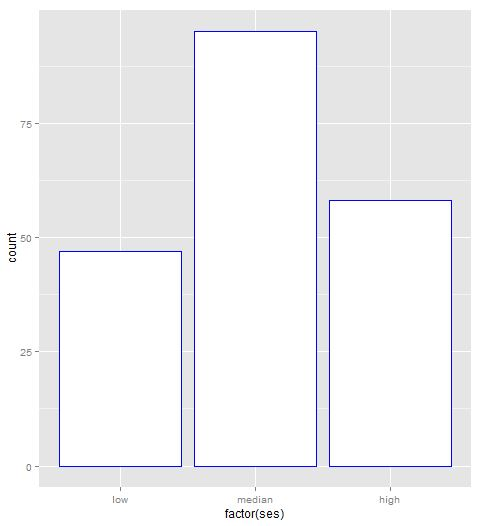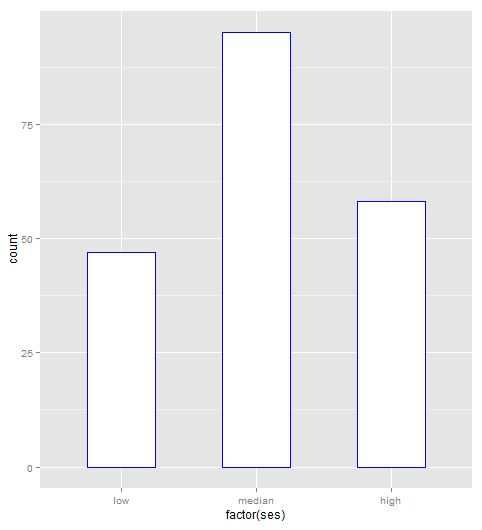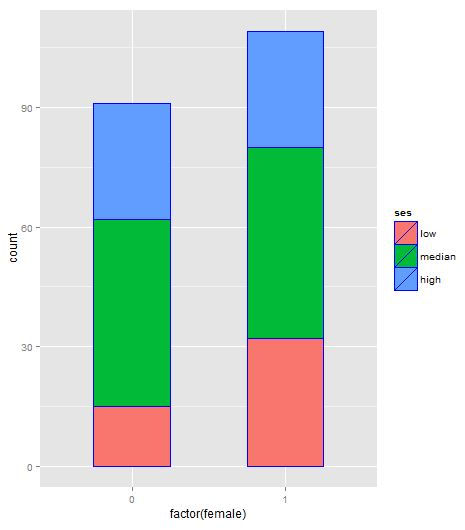## Sunday, December 9, 2012

### R: Replicate plot with ggplot2 (part 4) bar chart

The original plot from UCLA ATS is here. Here the same plot is done in package ggplot2.

Let's read in the data:
The bar chart here is to show how many observations for each level of ses(=1, 2 and 3 separately, will be replaced by "low", "median" and "high" separately).

The first plot is the plain bar chart:The second one is to replace the original value of ses by "low", "median" and "high" separately:The third one is to change the width of the bin.The forth is to group the data by female first, and then bin on each level of female(0 or 1). This is stacked plot. From the plot it is not easy to figure out which is higher. So we need to plot it separately as is shown in the fifth graph.The fifth one is plot bar chart at each level of female. That is, plot two bar chart for ses at 0 and 1 level of females separately.